# Digital Music Programming II: average~

This lab demonstrates how to receive and send audio signals in an MSP object and implementing a linear filter.

The averaging filter takes the average of two numbers, according to the equation:

```       average = (number1 + number2) / 2
```
To make a filter for averaging, the following difference equation can be used:
```      y[n] = (x[n] + x[n-1]) / 2
```
Where y is the output signal, x is the input signal, and n is the time index. In English, the averaging filter would be described thus: the current output is equal to the sum of the current input plus the last input which is then divided by 2. Here is an illustration of how the averaging filter works: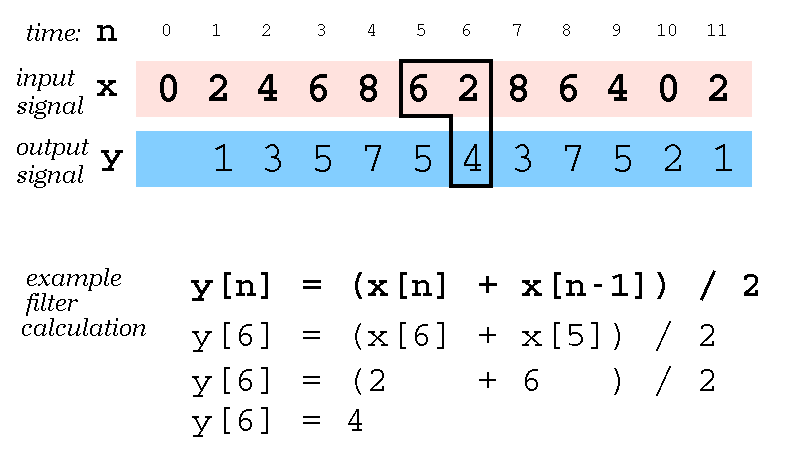The averaging filter can be described graphically as well. Here is a picture of the flowgraph for the filter which describes graphically how the input signal is processed to generate the output signal.If you put sinewaves with different frequencies into the averaging filter, they will be affected differently depending on the frequency of the sinewave. Try to calculate the output signals for the following special case discrete sinewaves:

 0 Hz (DC) 1, 1, 1, 1, 1, 1, 1, 1, 1, ... Nyquist frequency (fs/2) 1, -1, 1, -1, 1, -1, 1, -1, 1, ... 1/2 Nyquist frequency (fs/4) 1, 0, -1, 0, 1, 0, -1, 0, 1, ...
Here is a plot of how the amplitude of an input sinewave at various amplitudes will be affected by the averaging filter. The 0 Hz
 outputamplitude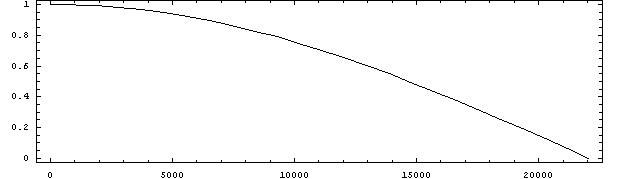frequency (sampling rate = 44,100 Hz)

If a DC signal is sent into the averaging filter, then it is not affected at all and the output amplitude will remain constant. On the other hand, if the input signal is at the Nyquist frequency (1/2 of the sampling rate), then there will be no signal output at all. If you try 1/2 of the Nyquist frequency, the output signal amplitude will be about 0.7 times the original input amplitude.

The averaging filter decreases the amplitude of sinewaves as the frequency of the sinewaves increases. Thus, the averaging filter is a low-pass filter because it passes low frequencies while removing higher frequencies.

 Source Code Example Usage average.c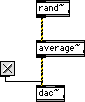``` #include "ext.h" #include "z_dsp.h" typedef struct { t_pxobject msp_data; float lastinput; float gain; } MyObject; void* object_data; void main (void); void* create_object (void); void MessageDSP (MyObject* mo, t_signal** signal, short* count); void MessageClear (MyObject* mo); t_int* Perform (t_int *parameters); void main(void) { setup((t_messlist**)&object_data, (method)create_object, (method)dsp_free, (short)sizeof(MyObject), NULL, A_NOTHING); addmess((method)MessageDSP, "dsp", A_CANT, A_NOTHING); addmess((method)MessageClear, "clear", A_NOTHING); dsp_initclass(); } void* create_object(void) { MyObject *mo = (MyObject*)newobject(object_data); dsp_setup((t_pxobject*)mo, 1); outlet_new((t_pxobject*)mo, "signal"); mo->gain = 0.5; return mo; } void MessageDSP(MyObject* mo, t_signal** signal, short* count) { #pragma unused(count) dsp_add(Perform, 5, 5, mo, signal->s_vec, signal->s_vec, signal->s_n); } void MessageClear(MyObject *mo) { mo->lastinput = 0.0; } t_int* Perform(t_int *parameters) { long pcount = (long) (parameters); MyObject *mo = (MyObject*)(parameters); t_float *input = (t_float*) (parameters); t_float *output = (t_float*) (parameters); long count = (long) (parameters); long i; for (i=0; ilastinput) * mo->gain; mo->lastinput = input[i]; } return parameters+pcount+1; } ```

# Exercises

1. Compile and test the average~ object. Test the averaging filter with different sinewaves and/or whitenoise as the input.

2. Create a difference~ object which uses the following difference equation:
```   y[n] = (x[n] - x[n-1]) / 2
```
Listen to the output of the difference~ filter and describe how it is different from the average~ filter.

3. Create a Max/MSP patch like the following: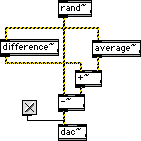Describe the output sound.

4. Create the following Max/MSP patch: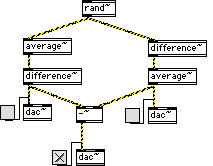Connect only one dac~ at a time (or you will hear all dac~ inputs at the same time). Listen to the output at the three indicated areas. Does the left and right sound outputs sound different or the same? Described the sound for the bottom output.